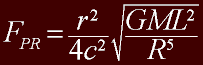This calculator computes the Poynting-Robertson force on an object, where:

• FPR is the Poynting-Robertson force.
• r is the radius of the object.
• G is the gravitational constant.
• M is the mass of the object.
• L is luminosity of the light source.
• R is the distance between the object and the light source.